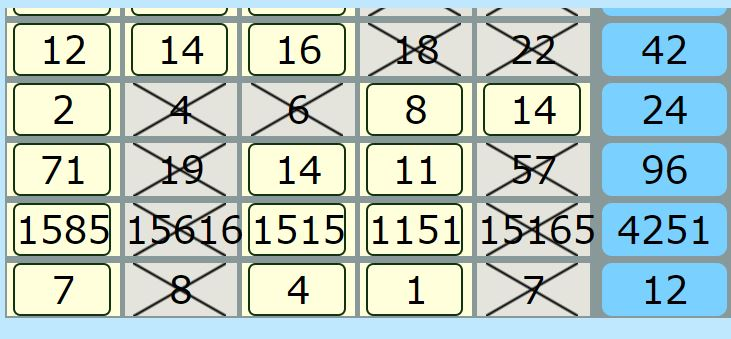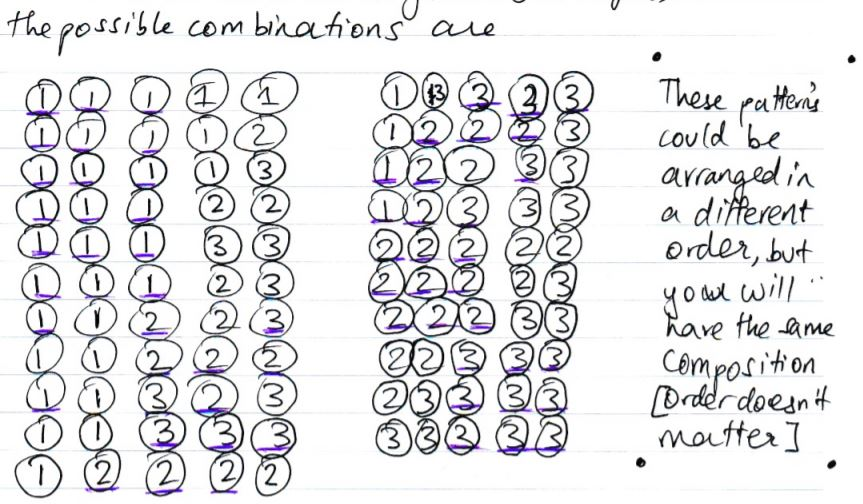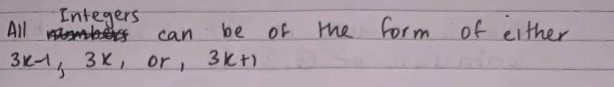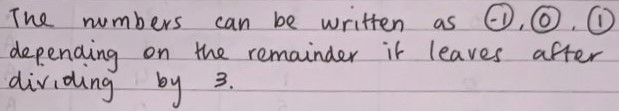#### You may also like### Calendar Capers

Choose any three by three square of dates on a calendar page...Make a set of numbers that use all the digits from 1 to 9, once and once only. Add them up. The result is divisible by 9. Add each of the digits in the new number. What is their sum? Now try some other possibilities for yourself!### Doodles

Draw a 'doodle' - a closed intersecting curve drawn without taking pencil from paper. What can you prove about the intersections?

# Take Three from Five

##### Age 11 to 16Challenge Level

Miraya from Heckmondwike Grammar School in the UK wrote:

According to all numbers I have tried, I think it is not possible to find a set of five numbers that do not have a set of three that sum up to a multiple of three.Samuel from Seely Primary School in England, Jimin (James) from BSKL in Malaysia, Harvey from St Nicholas in the UK, Ekaansh from Dulwich College Beijing in China, Kai and Wesley from Wilson's School in England, Ariel from Diocesan Girls' School in Hong Kong and Aditya from Bishop Vesey's Grammar School in the UK used the idea of remainders when dividing by 3. Ekaansh wrote:

I do not think this is possible. This is because any integers we pick would be one of these:

• Exactly divisible by 3 e.g. 3,6,9 etc.
• Multiple of 3 plus remainder 1 e.g. 4, 7, 10 etc.
• Multiple of 3 plus remainder 2 e.g. 5, 8, 11 etc.

Harvey represented the numbers in a different way, but the idea the same:

You can have three groups of numbers: multiples of three minus 1, multiples of three, and multiples of three plus one. If you continuously add or subtract three you can get one of three numbers: 1, 2, or 3.

Jimin (James) continued:

We only have to add up these [remainders] (1-2-0) because if they add up and make a number which is a multiple of 3, the left over 3s in the number will make a multiple of 3 anyways.

Samuel exaplined this idea using letters for the types of number:

I represented the numbers that are one more than a multiple of three as $x$, numbers that are 2 more than a multiple of 3 as $y$, and numbers that are a multiple of 3 as $z$.

$x$+$y$+$z$= a multiple of 3.
Example: 1+2+3=6

$z$+$z$+$z$=a multiple of 3
Example: 3+3+3=9

$y$+$y$+$y$=a multiple of 3
Example: 2+2+2=6

$x$+$x$+$x$=a multiple of 3
Example: 1+1+1=3

Since every number has to be either $x$, $y$ or $z$, and that these combinations work, it is impossible to produce a set of 5 whole numbers that any 3 of them do not add up to a multiple of 3.

This is because in a group of 5, it is not possible to have no groups of 3 [of the same type], whilst also not having one of each type.

This is Ariel's explanation of why there will always be three numbers that add up to a multiple of 3:

First, if there is at least 3 of a kind, there must be 3 integers that add up to a multiple of 3 because any 3 of a kind sums up to a multiple of 3.

Secondly, if there is no 3 of a kind, the combination of the remainders must be 2, 2, 1, in any order. In that case,there must be 3 integers that add up to a multiple of 3 since 0+1+2 sums up to a multiple of 3.

Therefore, there must be 3 integers that add up to a multiple of 3 no matter what 5 integers you choose.

To be absolutely sure that it's impossible, Aditya found all of the possible sets of remainders that 5 numbers could have:This idea of using remainders is very useful in number theory and is called modular arithmetic.

Emily from Garden International School in Malaysia, Rin from Wren Academy in England, Mahdi from Mahatma Gandhi International School in India and Sunhari all used the same idea, but expressed the numbers using algebra. This is Emily's work:

[All] integer[s] can be divided in three groups which are $3n,$ $3n+1$ or $3n+2$. $n$ can be any whole number.

We have 2 [ways of making a multiple of 3]

The 1st option is we have at least one number from each group. We can use the letters $n,$ $m$ and $l$ to show the different numbers. So when we add up the numbers it'll look something like this, $$3n+(3m+1)+(3l+2)= 3(n+m+l)+3= 3(n+m+l+1)$$As you can see this number is definitely a multiple of $3$ because we can look at the
numbers in the bracket as [an] integer and $3$ has to multiply that number. Therefore $3(n+m+l+1)$ can be divided by $3$.

Note that $3n, 3n+1$ and $3n+2$ represent a multiple of $3$, the number $1$ more than that multiple of $3$ and the number $2$ more than that multiple of $3$. That is why it is important to use $m$ and $l$ as well as $n$.

The second option is we have at least three numbers from one group.

Sunhari showed this option algebraically:

$(3t+1) + (3j+1) + (3w+1)$
$= 3t + 1 + 3j + 1 + 3w + 1$
$= 3(t+j+w) + 3$
Therefore, it would be a multiple of $3$.

$(3x+2) + (3g+2) + (3d+2)$
$= 3x + 2 + 3g + 2 + 3d + 2$
$= 3(x+g+d) + 6$
Therefore, it would be a multiple of $3$.

Rin explained why this means that there will always be three numbers that add up to a multiple of three.

1. If there are three or more multiples of $3$ within the five given numbers, we can add those to make a multiple of $3.$

2. If there are one or two multiples of $3$, other three numbers must be either $3n+1$ or $3n+2.$ If the remaining numbers include:
1. Three or more $3n+1$ s
We can add these three to get a multiple of 3.
2. Three or more $3n+1$ s
Similarly, we can add these to get a multiple of 3.
3. Otherwise
We can pick one each from groups of $3n, 3n+1, 3n+2$ and add together.

3. If there is no multiple of 3, the five numbers can be written as either $3n+1$ or $3n+2.$
For any combination of five numbers, three or more must be in the form of $3n+1$ or $3n+2.$
Adding numbers in the same group would give a multiple of $3.$

Therefore for every combination of five numbers, there are three numbers that add to give a multiple of $3.$

Mahdi used the same idea, but with $-1$ instead of $2$. Everything else works out the same.